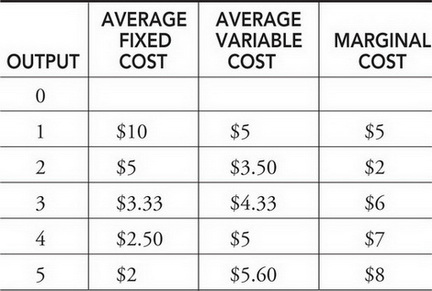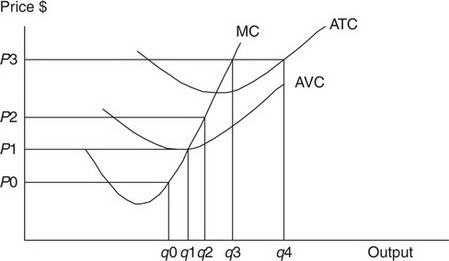# AP Microeconomics Practice Test 12

### Test Information12 questions14 minutes

1. Question below is based on the table of costs below for a perfectly competitive firm.The total fixed cost of producing a quantity of 4 is

2. Question below is based on the table of costs below for a perfectly competitive firm.The first unit of output to exhibit diminishing marginal productivity is the _____ unit.

3. Question below is based on the table of costs below for a perfectly competitive firm.At a quantity of 4, what is the total cost of production?

4. Monopolistic competition is often characterized by

5. The Figure below illustrates the short-run cost curves of a perfectly competitive firm.The shutdown point is seen at:

6. The Figure below illustrates the short-run cost curves of a perfectly competitive firm.If the market price of the output increases from P1 to P3, the profit-maximizing firm will

7. If the perfectly competitive price is currently below minimum average total cost, we can expect which of the following events in the long run?

8. When a monopolist has maximized profit,

9. Which of the following is necessarily a characteristic of oligopoly?

10. The market structures of perfect competition and monopolistic competition share which of the following characteristics?

11. If the government wishes to regulate a natural monopoly so that it produces an allocatively efficient level of output, it would be at an output

12. Which of the following is most likely to decrease the demand for kindergarten teachers?